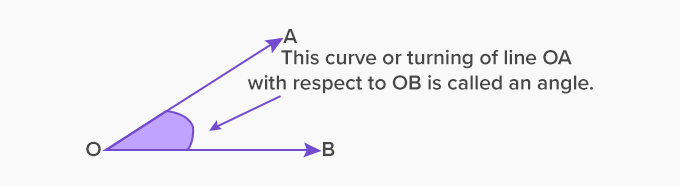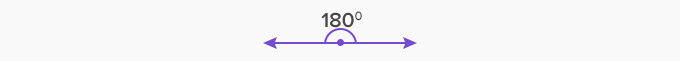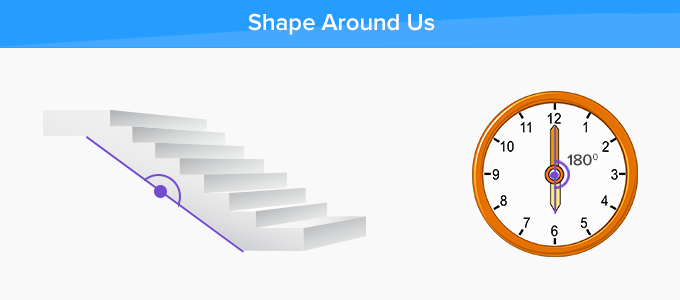# Straight Angle - Definition with Examples

The Complete K-5 Math Learning Program Built for Your Child

• 40 Million Kids

Loved by kids and parent worldwide

• 50,000 Schools

Trusted by teachers across schools

• Comprehensive Curriculum

Aligned to Common Core

What is Straight Angle?

Two joining rays make an angle. The common point where these two rays meet is called the vertex and the rays are called arms of the angle.

The measure of an angle is the amount of turn or rotation of a point from one arm to another along its vertex.When the arms of the angle lie in the opposite direction, they form a straight angle. The angles make a straight line through the vertex.

A straight angle is also called ‘flat angle’.In the picture, OX and OY are the arms of an angle. The common point O where the rays meet is called the vertex.

How to represent a straight angle?

There are various ways to represent straight angles as per the measurement system.

1. It is written as 180°

2. It is also written as π

All angles given in the picture are straight angles.Properties of a straight angle

• A straight angle is formed by rotating one ray by 180°with respect to another ray.• In a straight angle, the angle rays face in the opposite directions.

• A straight angle reverses the direction of a point

• A straight angle is exactly half of a revolution.

• A straight angle can also be formed by adding two right angles.

Drawing a straight angle using a protractor

1. Draw a straight-line OA, with an arrowhead at A.

2. Now keep the protractor on this line such that the baseline of the protractor is aligned over OA. The A should be pointing towards the 0°of the protractor.

3. Start from 0° and move towards 180° marking of the protractor. On arriving at 180°, mark a point B on the paper.

4. Join the vertex of the line OA with the point B. the second ray OB will have the arrowhead at B.Straight angles in real life

• The inclined plane of a staircase represents a straight angle.

• The straight angle of a see-saw.Fun Facts Straight angles look like a straight line, but it has two arms. On the other hand, a straight line has only one arm that points in one direction.

Won Numerous Awards & Honors